• 设X,Y相互独立，均服从[0，1]上的均匀分布，求Z=X+Y的分布设X,Y相互独立，均服从[0，1]上的均匀分布，求Z=X+Y的分布 设X,Y相互独立，均服从[0，1]上的均匀分布，求Z=X+Y的分布 分布函数法 z<0,Fz(z)=00≤z<1,...
$设X,Y相互独立，均服从[0，1]上的均匀分布，求Z=X+Y的分布$分布函数法
$z<0,F_z(z)=0\\ 0 \leq z <1,F_z(z)=\int_0^zdx \int_0^{z-x} f(x,y)dy\\ 0 \leq z <1,F_z(z)=1-\int_{z-1}^1dx \int_{z-x}^1 f(x,y)dy\\ 算完分段函数后再分段求导即可得f_z(z)$
换元法
$由公式f_z(z)=\int_{-\infty}^{+\infty}f(z-y,y)dy\\ 难点在于换元后,积分限的确定(如果是直角换极坐标的情况好一点)\\ y\in (0,1),x=z-y\in (0,1) \rightarrow y\in(0,1) 并(z-1,z)\\ y\in \begin{cases} (0,z)& \text{0=1} \end{cases}\\ f_z(z)= \begin{cases} \int_{0}^{z}f(z-y,y)dy& \text{0=1} \end{cases}\\$
方法论：一般地，对于无穷区间，直接换好，其它的情况先积后导


展开全文• 通过建立二维随机变量(X,Y)的线性组合αX+βY的概率密度函数的推导公式,给出了在可靠性工程中应用最广泛的二维指数分布的线性组合的概率分布.
• 该文章介绍三种离散型随机变量及三种连续性随机变量。 一、离散型随机变量 1.项分布 n重伯努利实验中，A发生的次数服从二项分布...、连续型随机变量 1.均匀分布 1）密度函数 2）表示 2.指数分布 1）分布函数 ...
该文章介绍三种离散型随机变量及三种连续性随机变量。
一、离散型随机变量
1.二项分布
n重伯努利实验中，A发生的次数服从二项分布，其中p表示每次实验中A发生的概率，比如抛n次硬币正面出现的次数。
1）概率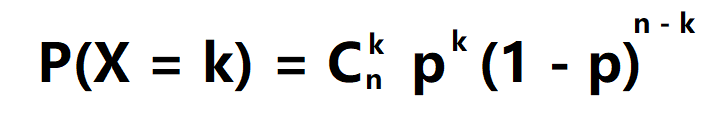2）表示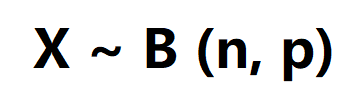2.Poisson 分布
1）概率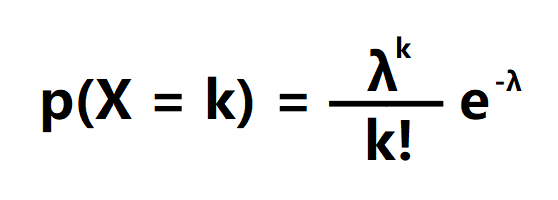2）表示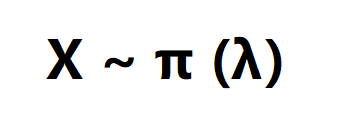3.几何分布
1）概率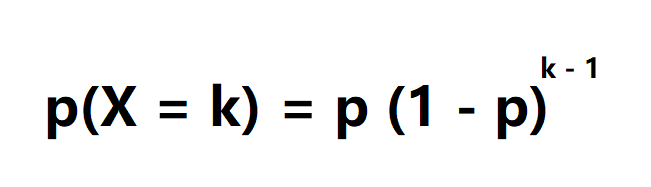2）表示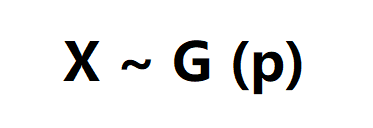二、连续型随机变量
1.均匀分布
1）密度函数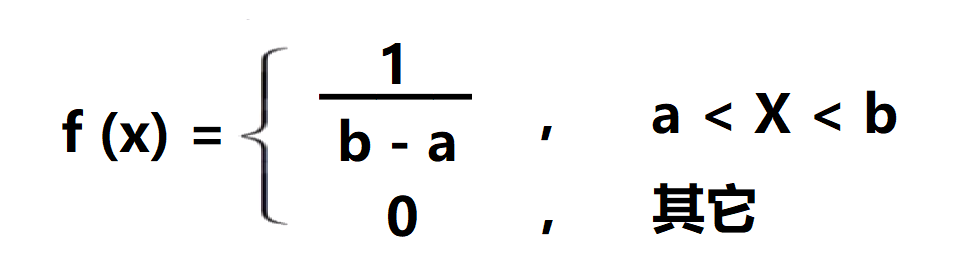2）表示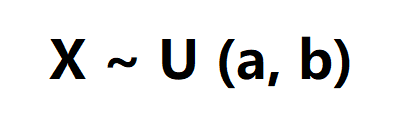2.指数分布
1）分布函数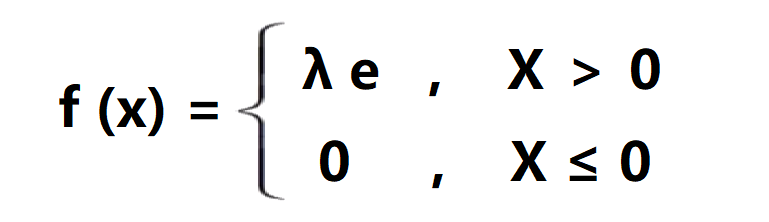2）表示3.正态分布
1）分布函数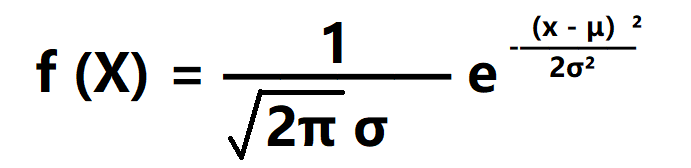2）表示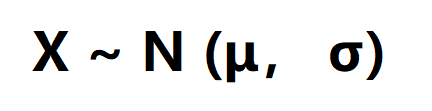展开全文统计学
• 答： 均匀分布的概率密度函数为： 因为a,b独立，所以二维随机变量(a,b)的概率密度函数为： 于是根据期望值公式可以得到： 又有: （1）当a>b时， （2）当b>a时， 综上所述： ...
答：
均匀分布的概率密度函数为：因为a,b独立，所以二维随机变量(a,b)的概率密度函数为：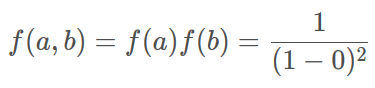于是根据期望值公式可以得到：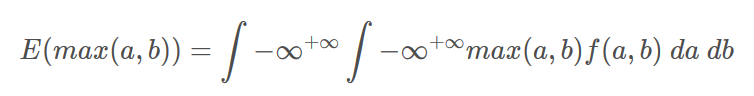又有:
（1）当a>b时，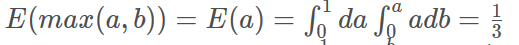（2）当b>a时，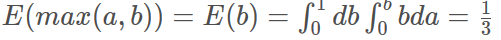综上所述：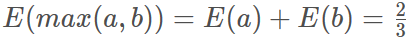展开全文• 答： 均匀分布的概率密度函数为： f(x)={1b−a,a<x<b0,else f(x) = \begin{cases} \frac{1}{b-a}, & a<x<b \\ ...因为a,b独立，所以二维随机变量(a,b)的概率密度函数为： f(a,b)=...
答：
均匀分布的概率密度函数为：
$f(x) = \begin{cases} \frac{1}{b-a}, & a
因为a,b独立，所以二维随机变量(a,b)的概率密度函数为：
$f(a,b)=f(a)f(b)=\frac{1}{(1-0)^2}$
于是根据期望值公式可以得到：
$E(max(a,b))=\int{-\infty}^{+\infty} \int{-\infty}^{+\infty} max(a,b)f(a,b)\ da\ db$
又有:
（1）当a>b时，$E(max(a,b))=E(a)=\int_0^1da \int_0^aadb=\frac{1}{3}$
（2）当b>a时，$E(max(a,b))=E(b)=\int_0^1db \int_0^bbda=\frac{1}{3}$
综上所述：
$E(max(a,b))=E(a)+E(b)=\frac{2}{3}$


展开全文• 若干个随机变量放在一起形成的多变量总体，一个形如的p向量，其中X1到Xn服从正态分布。 .随机向量的分布： 联合分布，边缘分布，条件分布（与概率论定义一致）。 三.数字特征： 均值向量： 协方差阵： ...
• 一元正态分布回顾如果随机变量 服从均值为 方差为 的正态分布 (Univariate normal distribution)， ，则其概率密度函数为：整个分布可以仅用均值及...若 维随机变量 服从均值向量为 和协方差矩阵为 的多元正态分布 ...
• 1. 定义：设二维连续型随机变量（X，Y)的联合概率密度为 其中μ1，μ2，σ1，σ2，ρ均为常数，且σ1>0, σ2>0, |ρ|<1则称（X，Y）服从参数为μ1，μ2，σ1，σ2，ρ的二维正态分布。 记作（X，Y）~N...
• 书的购买链接书的勘误，优化，源代码资源概率密度函数是概率论中的核心概念之一，用于描述连续型随机变量服从的概率分布。在机器学习中，我们经常对样本向量x的概率分布进行建模，往往是连...
• 一元正态分布回顾如果随机变量 服从均值为 方差为 的正态分布 (Univariate normal ...正态分布可以模拟大量自然现象多元正态分布多元正态分布密度函数类比于一元情况，若 维随机变量 服从均值向量为 和协方差矩阵为 ...
• ①随机过程的任意n维随机变量服从高斯分布，则 就是高斯过程。 宽平稳高斯过程一定是严平稳的 ②若平稳高斯过程在任意两个时刻是不相关的，那么一定是互相独立的。 ③白噪声 三章 系统对随机信号的响应 （1）...
• 一、离散型的概率密度函数与累积密度函数对于一个离散型的随机变量 ，它可能服从我们已知的一些理论分布，也可能服从某个未知的乱七八糟的分布。这时候，我们有一种稳（cu）健（bao）的方式去描述它的分布情况，那...
• 1.一个宽平稳过程不一定是严...例2：服从柯西分布的随机变量序列是严平稳随机过程，但不是宽平稳随机过程。 2.宽平稳过程定只涉及与一维、二维分布有关的数字特征，所以一个严平稳过程只要二阶矩存在，则必定是宽平
• ## 高斯随机过程

千次阅读 2019-07-28 23:10:23
高斯过程：随机过程kesei(t)的任意n维分布服从正态分布，则称它为高斯过程，也就是说自变量满足正态分布。高斯分布不一定是平稳分布，要判断高斯过程是不是平稳过程，还要判断一维和二维，和之前判断一样的。 书上...通信原理
• 第一步：从平均分布到高斯分布 ...假设有两个独立随机变量X, Y服从高斯分布： 若在二维平面上采样横纵坐标分别为(X, Y)的点，得到的图为： 点(X, Y)在该平面上的联合概率密度函数为： 将平...
• 01-独立同分布 指随机过程中，任何时刻的取值都为随机变量，如果这些随机变量服从同一分布，并且互相独立，那么这些随机变量是独立同分布。先说说独立这个概念。在预测德克萨斯州区域的房屋价值时，房屋样本x1和...
• 1.如何计算二维随机变量的联合分布函数？ 思路：首先分类讨论：离散型：对分布律进行求和——连续型：求出概率密度函数，正确定限，积分。其中，正确定限是连续型求解中极其容易犯错的地方 例题：随机变量（x,y）...
• 基本概念随机变量：是一个量化随机事件的函数，表示随机事件发生的所有可能结果的取值，通常用大写字母表示。如：概率分布：描述随机变量所有可能取值及其对应概率的统计图。. 常见的四个离散概率分布伯努利分布...
• 5．设二维随机变量（X，Y）的分布律为 Y X 1 2 3 1 2 则P{XY=2}=（ ） A． B． C． D． 6．设二维随机变量（X，Y）的概率密度为 则当 时，（X，Y）关于X的边缘概率密度为fx(x)=（ ） A． B．2x C． D...
• ## 正态分布

千次阅读 2016-11-25 16:38:15
则这个随机变量就称为正态随机变量，正态随机变量服从的分布就称为正态分布，记作   2. N维空间正太分布   N维空间正太分布方程为：    r为模糊半径。  于是，二维高斯模板上的距离中